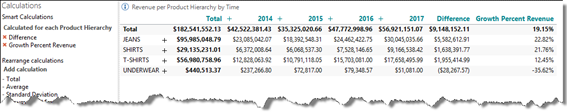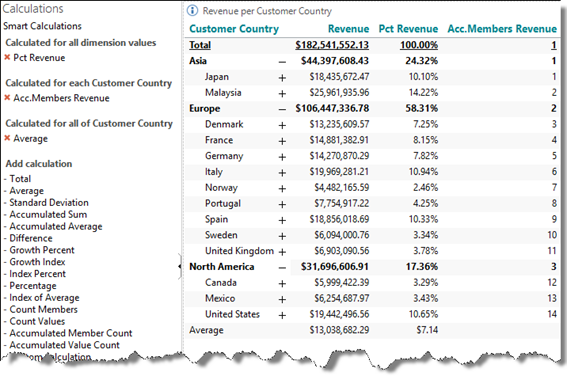# Pre-defined calculations

The list of pre-defined calculations, called Smart calculations, is found on Smartpad Calculations.

The pre-defined calculations has been designed, in an intelligent manner, to add as much value as possible in relation to the current context. In the example below, we have added a Difference and a Growth percent calculation to a crosstab with dimensions on both axes.Another example, a crosstab with a dimension on just one axis, shows how the Average, Percent and Accumulated members count will behave when added as Smart calculations.